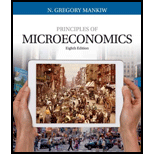# Xavier opens up a lemonade stand for two hours. He spends $10 for ingredients and sells$60 worth of lemonade. In the same two hours, he could have mowed his neighbor's lawn for $40. Xavier has an accounting profit of ________ and an economic profit of _______. a.$50, $10 b.$90, $50 c.$10, $50 d.$50, $90BuyFindarrow_forward ### Principles of Microeconomics (Mind... 8th Edition N. Gregory Mankiw Publisher: Cengage Learning ISBN: 9781305971493 #### Solutions Chapter SectionBuyFindarrow_forward ### Principles of Microeconomics (Mind... 8th Edition N. Gregory Mankiw Publisher: Cengage Learning ISBN: 9781305971493 Chapter 13, Problem 1CQQ Textbook Problem 192 views ## Xavier opens up a lemonade stand for two hours. He spends$10 for ingredients and sells $60 worth of lemonade. In the same two hours, he could have mowed his neighbor's lawn for$40. Xavier has an accounting profit of ________ and an economic profit of _______.a. $50,$10b. $90,$50c. $10,$50d. $50,$90

To determine
Accounting profit and economic profit.

Option ‘a’ is correct.

### Explanation of Solution

Option (a):

When Xavier spend $10 for ingredients and sell$60 worth of lemonade, then the accounting profit will be (Total revenueTotal explicit cost) (total revenue – total explicit cost) $50($60$10). Economic profit is (Total revenue(Implicit cost +Explicit cost))$10 ($60($50+$10)) . Therefore, the value of accounting profit is$50 and the economic profit is $10. Option (b): Xavier accounting profit is not$90, because an accounting profit is the total revenue minus the total explicit cost. Economic profit is not $50, because economic profit is total revenue minus implicit and explicit cost. Thus, option ‘b’ is incorrect. Option (c): When Xavier spends$10 for ingredients and sells $60 worth of lemonade, Xavier accounting profit will not be$10, because an accounting profit is the total revenue minus the total explicit cost. Economic profit is not $50, because economic profit is the total revenue minus implicit and explicit cost. Thus, option ‘c’ is incorrect. Option (d): When Xavier spend$10 for ingredients and sell $60 worth of lemonade, then the accounting profit will be (total revenue – total explicit cost)$50($60$10) . But the economic profit is not \$90, because the economic profit is (Total revenue(Implicit cost +Explicit cost)) . Thus, option ‘d’ is incorrect.

Economics Concept Introduction

Concept introduction:

Accounting profit: Accounting profit refers to the total revenue minus total explicit cost

Economic profit: Economic profit refers to the total revenue minus implicit and explicit cost.

#### The Solution to Your Study Problems

Bartleby provides explanations to thousands of textbook problems written by our experts, many with advanced degrees!

Get Started

Find more solutions based on key concepts
Should a managerial accounting system provide both financial and nonfinancial information? Explain.

Managerial Accounting: The Cornerstone of Business Decision-Making

Explain how payroll fraud can be prevented.

College Accounting (Book Only): A Career Approach

PREFERRED STOCK RETURNS Bruner Aeronautics has perpetual preferred stock outstanding with a par value of 100. T...

Fundamentals of Financial Management, Concise Edition (with Thomson ONE - Business School Edition, 1 term (6 months) Printed Access Card) (MindTap Course List)